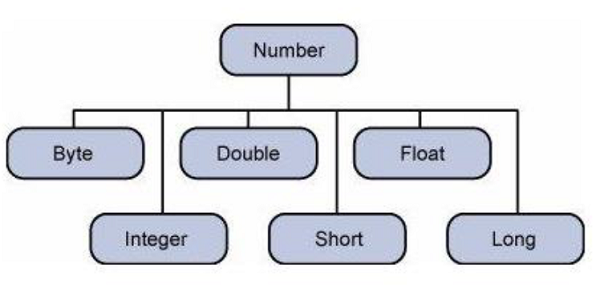# Clojure - Numbers

Numbers datatype in Clojure is derived from Java classes.

Clojure supports integer and floating point numbers.

• An integer is a value that does not include a fraction.

• A floating-point number is a decimal value that includes a decimal fraction.

Following is an example of numbers in Clojure.

```(def x 5)
(def y 5.25)
```

Where ‘x’ is of the type Integer and ‘y’ is the float.

In Java, the following classes are attached to the numbers defined in Clojure.To actually see that the numbers in Clojure are derived from Java classes, use the following program to see the type of numbers assigned when using the ‘def’ command.

## Example

```(ns clojure.examples.hello
(:gen-class))

;; This program displays Hello World
(defn Example []
(def x 5)
(def y 5.25)
(println (type x))
(println (type y)))
(Example)
```

The ‘type’ command is used to output the class associated with the value assigned to a variable.

## Output

The above code will produce the following output.

```Java.lang.long
Java.lang.double
```

## Number Tests

The following test functions are available for numbers.

Sr.No. Numbers & Description
1 zero?

Returns true if the number is zero, else false.

2 pos?

Returns true if number is greater than zero, else false.

3 neg?

Returns true if number is less than zero, else false.

4 even?

Returns true if the number is even, and throws an exception if the number is not an integer.

5 odd?

Returns true if the number is odd, and throws an exception if the number is not an integer.

6 number?

Returns true if the number is really a Number.

7 integer?

Returns true if the number is an integer.

8 float?

Returns true if the number is a float.